# Class 12 Maths NCERT Solutions for Chapter 9 Differential Equations Exercise 9.6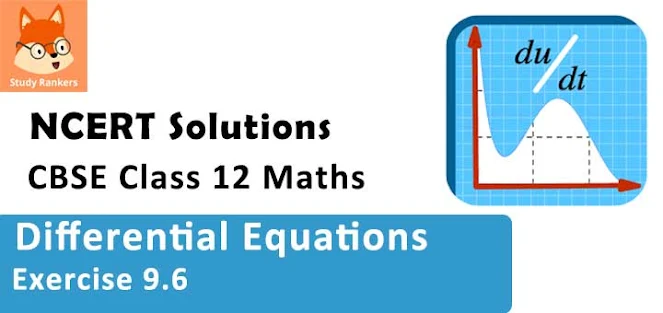### Differential Equations Exercise 9.6 Solutions

1. For the differential equation find the general solution :
dy/dx + 2y = sin x

Solution

The given differential equation is dy/dx + 2y = sin x
This is in the form of dy/dx + py = Q (where p = 2 and Q = sin x)
Now, I.F = e∫pdx = e∫3dx  = e3x
The solution of the given differential equation is given by the relation,
y(I.F.) = ∫(Q × I.F.) dx  + C
⇒ ye2x = ∫sin x. e2x dx + C ...(1)
Let I = ∫sin x. e2x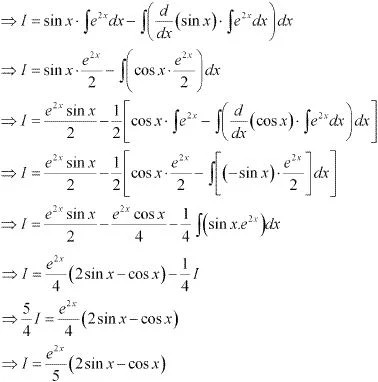Therefore, equation (1) becomes:
ye2x = e2x/5 (2 sin x - cos x) + C
⇒ y = 1/5 (2 sin x - cos x) + Ce-2x
This is the required general solution of the given differential equation.

2. For the differential equations find the general solution:
dy/dx + 3y = e-2x

Solution

The given differential equation is dy/dx + py = Q (where p = 3 and Q = e-2x)
Now, I.F = e∫pdx = e∫3dx = e3x
The solution of the given differential equation is given by the relation,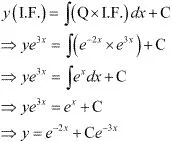This is the required general solution of the given differential equation.

3. For the differential equations find the general solution :
dy/dx + y/x = x2

Solution

The given differential equation is:
dy/dx + py = Q (where p = 1/x and Q = x2)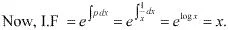The solution of the given differential equation is given by the relation,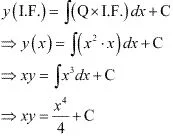This is the required general solution of the given differential equation.

4. For the differential equations find the general solution :
dy/dx + sec xy = tan x(0 ≤ x < π/2)

Solution

The given differential equation is :
dy/dx + py = Q  (where p = sec x and Q = tan x)
Now, I.F = e∫pdx = e∫secx dx = elog(secx + tanx) = sec x + tan x.
The general solution of the given differential equation is given by the relation,
y = (I.F.) = ∫(Q × I.F.) dx + C
⇒ y(sec x + tan x) = ∫tan x(sec x + tan x)dx + C
⇒ y(sec x + tan x) = ∫sec x tan x dx + ∫tan2 x dx + C
⇒ y(sec x + tan x) = sec x + ∫(sec2 x - 1)dx + C
⇒ y(sec x + tan x) = sec x + tan x - x + C

5. For the differential equations find the general solution :
cos2 x (dy/dx) + y = tan x(0 ≤ x < π/2)

Solution

The given differential equation is :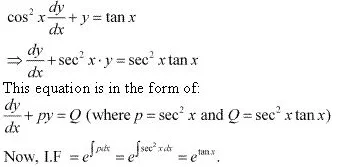The general solution of the given differential equation is given by the relation,
y(I.F.) = ∫(Q × I.F.) dx + C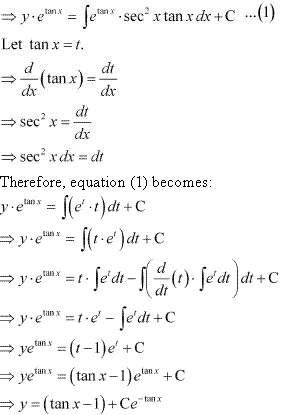6. For the differential equations find the general solution:
x(dy/dx) + 2y = x2 log x

Solution

The given differential equation is: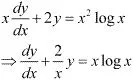This equation is in the form of a linear differential equation as :
dy/dx + py = Q (where p = 2/x and Q = x log x)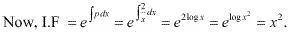The general solution of the given differential equation is given by the relation,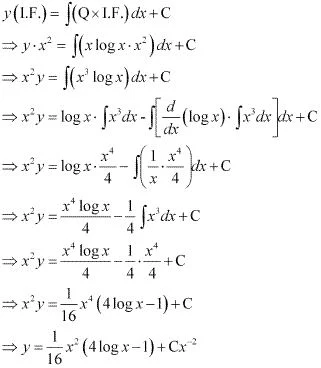7. For the differential equations find the general solution:
x log x(dy/dx) + y = (2/x) log x

Solution

The given differential equation is :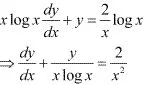This equation is the form of a linear differential equation as :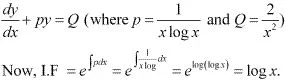The general solution of the given differential equation is given by the relation,
y(I.F.) = ∫(Q× I.F.)dx + C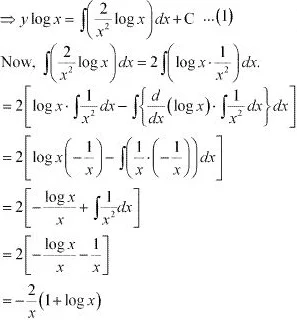Substituting the value of ∫[(2/x2)log x] dx in equation (1), we get :
y log x = (-2/x) (1 + log x) + C
This is the required general solution of the given differential equation.

8. For the differential equations find the general solution: (1 + x2) dy + 2xy dx = cot x dx (x ≠ 0).
Solution
(1 + x2 )dy + 2xy dx = cot x dx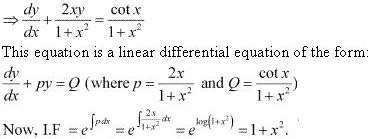The general solution of the given differential equation is given by the relation,
y(I.F.) = ∫(Q× I.F.) dx + C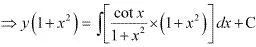⇒ y.(1+ x2) = ∫cot x dx + C
⇒ y (1 + x2) = log |sin x| + C

9. For the differential equations find the general solution:
x(dy/dx) + y - x + xy cot x = 0 (x ≠ 0)
Solution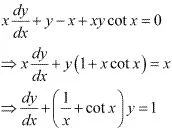This equation is a linear differential equation of the form :
dy/dx + py = Q  (where p = 1/x + cot x and Q = 1)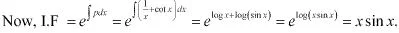The general solution of the given differential equation is given by the relation,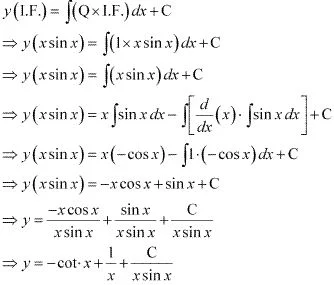10. For the differential equations find the general solution :
(x + y) dy/dx = 1
Solution
(x + y) dy/dx  1
⇒ dy/dx = 1/(x + y)
⇒ dx/dy = x + y
⇒ (dx/dy) - x = y
This is a linear differential equation of the form :
dy/dx + px = Q (where p = -1 and Q = y)
Now, I.F. =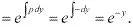The general solution of the given differential equation is given by the relation,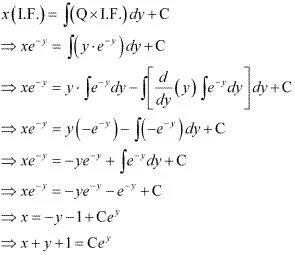11. For the differential equations find the general solution: y dx + (x – y2) dy = 0
Solution
y dx + (x - y2)dy = 0
⇒ y dx = (y2 - x)dy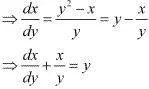This is a linear differential equation of the form :
dy/dx + px = Q (where p = 1/y and Q = y)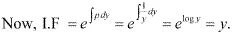The general solution of the given differential equation is given by the relation,
x(I.F.) = ∫(Q × I.F.) dy + C
⇒ xy = ∫(y.y) dy + C
⇒ x = ∫ y2 dy + C
⇒ xy = y3 /3 + C
⇒ x = y2/3 + C/y

12. For the differential equations find the general solution :
(x + 3y2 )(dy/dx) = y (y > 0)
Solution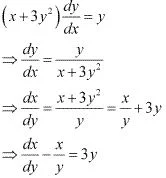This is a linear differential equation of the form :
dx/dy  + px = Q (where p = -1/y and Q = 3y)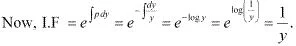The general solution of the given differential equation is given by the relation,
x(I.F.) = ∫(Q × I.F.)dy + C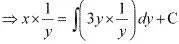⇒ x/y = 3y + C
⇒ x = 3y2 + Cy

13. For the differential equations given find a particular solution satisfying the given condition :  dy/dx + 2y tan x = sin x ; y = 0 when x = π/3
Solution
The given differential equation is dy/dx + 2y tan x = sin x.
This is a linear equation of the form:
dy/dx + py = Q (where p = 2 tan x and Q = sin x)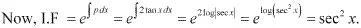The general solution of the given differential equation is given by the relation,
y(I.F.) =  ∫(Q × I.F.)dx + C
⇒ y(sec2 x) = ∫(sin x. sec2 x)dx + C
⇒ y sec2 x = ∫(sec x. tan x)dx + C
⇒ ysec2 x = sec x + C ...(1)
Now, y = 0 at x = π/3 .
Therefore,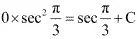⇒ 0 = 2 + C
⇒C = -2
Substituting C = -2 in equation (1), we get :
y sec2 x = sec x - 2
⇒ y = cos x - 2cos2 x
Hence, the required solution of the given differential equation is y = cos x - 2cos2 x

14. For the differential equations given find a particular solution satisfying the given condition :   (1 + x2)(dy/dx) + 2xy = 1/(1 + x2); y = 0 when x = 1
Solution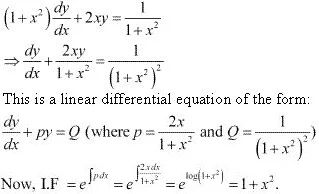The general solution of the given differential equation is given by the relation,
y(I.F.) = ∫(Q × I.F.) dx + C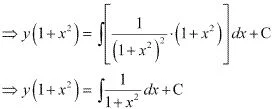⇒ y(1 + x2 ) = tan-1 x + C ...(1)
Now, y = 0 at x = 1.
Therefore,
0 = tan-1 1 + C
⇒ C = -π/4
Substituting C = -π/4 in equation (1), we get :
y(1 + x2) = tan-1 x - π/4
This is the required general solution of the given differential equation.

15. For the differential equations given find a particular solution satisfying the given condition : dy/dx - 3y cot x = sin 2x; y = 2 when x = π/2
Solution
The given differential equation is dy/dx - 3y cot x = sin 2x.
This is a linear differential equation  of the form :
dy/dx + py = Q (where p = -3 cot x and Q = sin 2x)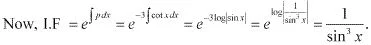The general solution of the given differential equation is given by the relation,
y(I.F.) = ∫(Q × I.F.) dx + C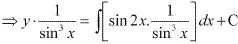⇒ y cosec3 x = 2 ∫(cot x cosec x) dx + C
⇒ y cosec3 x = 2 cosec x + C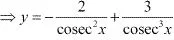⇒ y = -2sin2 x + C sin3 x ...(1)
Now, y = 2 at x = π/2
Therefore, we get:
2 = -2 + C
⇒ C = 4
Substituting C = 4 in equation (1), we get :
y = -2sin2 x + 4sin3 x
⇒ y = 4sin3 x - 2sin2 x
This is the required particular solution of the given differential equation.

16. Find the equation of the curve passing through the origin given that the slope of the tangent to the curve at any point (x, y) is equal to the sum of the coordinates of the point.
Solution
Let F(x, y) be the curve passing through the origin.
At point (x, y), the slope of the curve will be dy/dx.
According to the given information:
dy/dx = x + y
⇒ dy/dx - y = x
This is a linear differential equation of the form :
dy/dx + py = Q (where p = -1 and Q = x)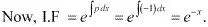The general solution of the given differential equation is given by the relation,
y(I.F.) = ∫(Q × I.F.) dx + C
⇒ ye-x = ∫xe-x dx + C ...(1)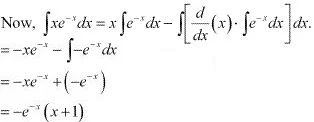Substituting in equation (1), we get :
ye-x = -e-x (x + 1) + C
⇒ y = -(x + 1) + Cex
⇒ x + y + 1 = Cex ...(2)
The curve passes through the origin .
Therefore, equation (2) becomes :
1 = C
C = 1
Substituting C = 1 in equation (2), we get :
x + y + 1 =ex
Hence, the required equation of curve passing through the origin is x + y + 1 = ex

17. Find the equation of a curve passing through the point (0, 2) given that the sum of the coordinates of any point on the curve exceeds the magnitude of the slope of the tangent to the curve at that point by 5.
Solution
Let F(x, y) be the curve and let (x, y) be a point on the curve. The slope of the tangent to the curve at (x, y) is dy/dx.
According to the given information:
(dy/dx) + 5 = x + y
⇒ dy/dx - y = x - 5
This is a linear differential equation of the form :
dy/dx + py = Q (where p = -1 and Q = x - 5)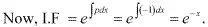The general equation of the curve is given by the relation,
y(I.F.) = ∫(Q × I.F.) dx + C
⇒ y . e-x = ∫(x- 5)e-x dx + C ...(1)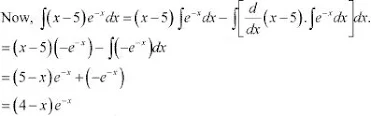Therefore, equation (1) becomes :
ye-x = (4 - x)e-x  + C
⇒ y = 4 - x + Cex
⇒ x + y - 4 = Cex ...(2)
The curve passes through point (0, 2).
Therefore, equation (2) becomes :
0 + 2 - 4 = Ce0
⇒ -2 = C
⇒ C = -2
Substituting C = -2 in equation (2), we get :
x + y - 4 = -2ex
⇒ y = 4 - x - 2ex
This is the required equation of the curve.

18. The integrating Factor of the differential equation (dy/dx) - y = 2x2 is
(A) e-x
(B) e-y
(C) 1/x
(D) x
Solution
The given differential equation is :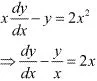This is a linear differential equation of the form :
dy/dx + py = Q (where p = -1/x and Q = 2x)
The integrating factor (I.F.) is given by the relation,
The
Hence, the correct answer is C.

19. The integrating factor of the differential equation .
(1 - y2) dx/dy + yx = ay(-1 << 1) is
Solution
The given differential equation is :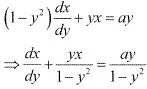This is a linear differential equation of the form :
dx/dy + py  = Q [where p = y/(1 - y2) and Q = ay/(1 - y2)]
The integrating factor (I.F.) is given by the relation ,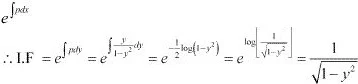Hence, the correct answer is D.